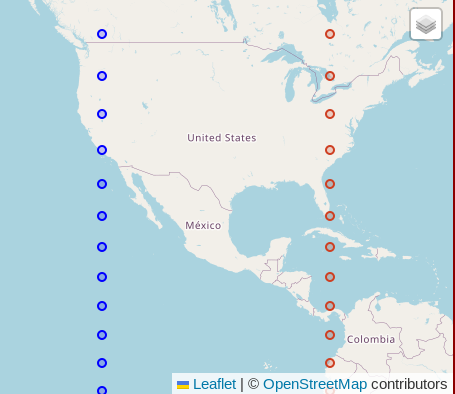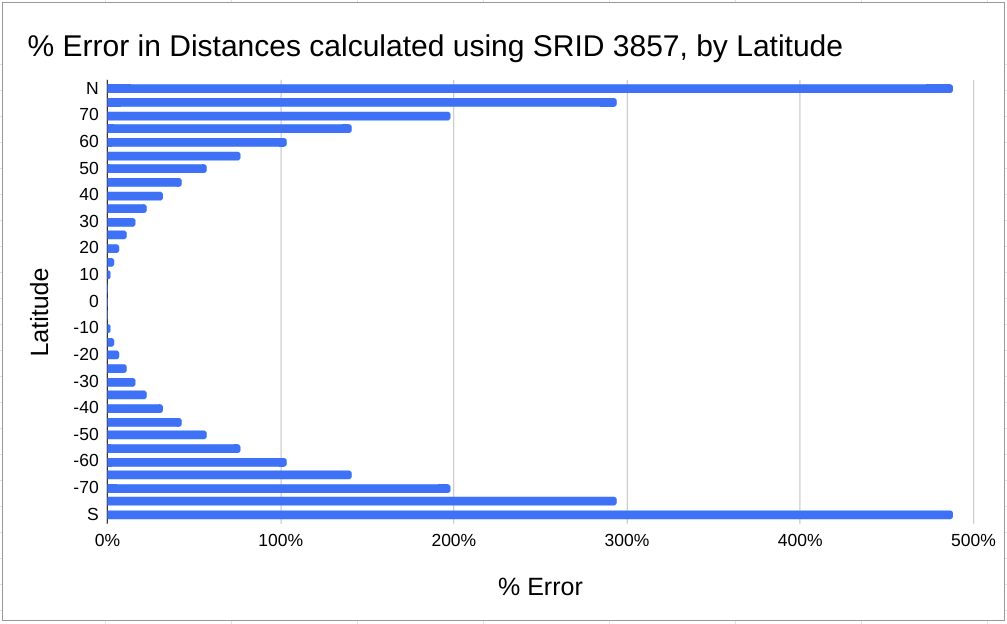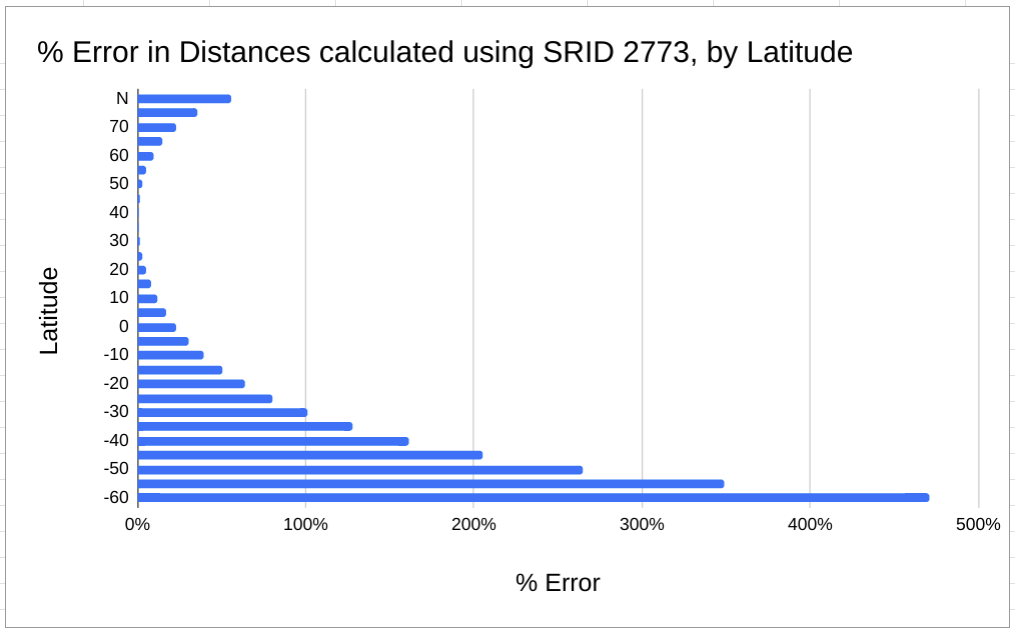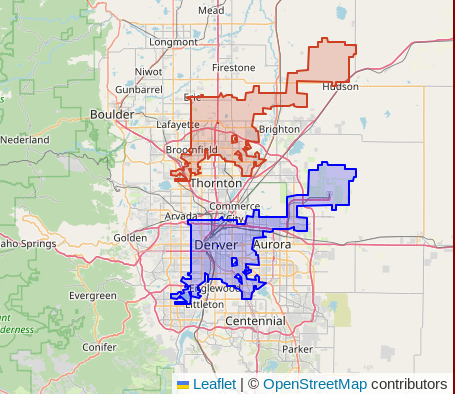My book Mastering PostGIS and OpenStreetMap is available!

# Accuracy of Geometry data in PostGIS

By Ryan Lambert -- Published April 15, 2023

A common use case with PostGIS data is to calculate things, such as distances between points, lengths of lines, and the area of polygons. The topic of accuracy, or inaccuracy, with `GEOMETRY` data comes up often. The most frequent offenders are generic SRIDs such as 3857 and 4326. In some projects accuracy is paramount. Non-negotiable. On the other hand, plenty of projects do not need accurate calculations. Those projects often rely on relationships between calculations, not the actual values of the calculations themselves. If Coffee shop Y is 4 times further away than Coffee shop Z. I'll often go to Coffee shop Z just based on that.

In most cases, users should still understand how significant the errors are. This post explores one approach to determine the how accurate (or not!) the calculations of a given SRID are in a particular region, based on latitude (North/South). The queries used in this post can be adjusted for your specific area.

## Set the stage

The calculations in this post focus on the distance of two points situated 40 decimal degrees apart. The points are created in pairs of west/east points at -120 (W) and -80 (W). Those were picked arbitrarily, though intentionally spread far enough apart to make the errors in distance calculations feel obviously significant. The point pairs are created in 5 decimal degree intervals of latitude from 80 North to 80 South. The following screenshot shows how the points frame in much of North America.

While the points on the map using a mercator projection appear to be equidistant... they are not!The code to replicate the data in this post is at the end under Queries to Explore.

## Accuracy by Latitude: SRID 3857

The following chart shows the percentage error in distance calculations with `GEOMETRY` data in SRID 3857. The error rate of SRID 3857 at the equator is basically zero (0.1%). At 60 degrees North/South the error rate exceeds 100%, meaning a 1 mile distance has grown to more than 2 miles.

The error rate is presented as a percent difference, calculated as `(distance 3857 - distance geography) / distance geography`.The following table shows a few of the raw numbers behind the latitude calculations. Notice that the distance of the geometry in SRID 3857 (`distance_3857_km`) is calculated as 4,452.77 km for each row. While that's only 0.1% off the real distance between the points at the equator (`lat = 0`), the distances using SRID 3857 incorrectly report that same value at all latitudes. At 40 degrees North/South, SRID 3857 is off by 32%. The error rate grows faster as the data moves closer to the poles, away from the equator.

``````┌─────┬───────────────────┬────────────────────┬───────────────────────┐
│ lat │ distance_3857_km  │  distance_geog_km  │     percent_error     │
╞═════╪═══════════════════╪════════════════════╪═══════════════════════╡
│  80 │ 4452.779631730942 │  757.2089865246866 │     4.880516093935412 │
│  40 │ 4452.779631730942 │ 3377.8673150206587 │    0.3182221847289195 │
│   0 │ 4452.779631730942 │  4447.803189385262 │ 0.0011188539901127623 │
│ -40 │ 4452.779631730942 │ 3377.8673150206587 │    0.3182221847289195 │
│ -80 │ 4452.779631730942 │  757.2089865246866 │     4.880516093935412 │
└─────┴───────────────────┴────────────────────┴───────────────────────┘
``````

## Using local SRIDs

You can get accurate calculations using `GEOMETRY` with localized SRIDs designed for your specific area. A while back I created the SRID Bounding Boxes project which makes it easy to find an appropriate SRID for any area on Earth. I wrote about using that project in this post, the data and code are available on GitHub. My book, Mastering PostGIS and OpenStreetMap, includes a section showing how to scale the SRID bounding boxes to use globally with the help of Postgres' generated columns.

Most of the PostGIS work I do is focused around Colorado with a significant portion of my queries focused around the Denver metro area. According to the OpenStreetMap point I have in my database, Denver, CO is at 39.74 degrees north. I regularly use SRID 2773 for geometry calculations in this region, so how accurate are those? The following chart and table shows that at 40 N the distance in the geometry calculation is only 0.3% (0.003127) off of the geography calculation.I chose to exclude values beyond 60 South in the above chart because their error rate is so extreme it made the chart an unusable visual.

``````┌─────┬────────────────────┬────────────────────┬───────────────────────┐
│ lat │  distance_2773_km  │  distance_geog_km  │     percent_error     │
╞═════╪════════════════════╪════════════════════╪═══════════════════════╡
│  80 │ 1181.1183183489434 │  757.2089865246866 │    0.5598313535208373 │
│  40 │ 3388.4302190583244 │ 3377.8673150206587 │ 0.0031270926453193446 │
│   0 │  5467.435324912753 │  4447.803189385262 │   0.22924398677550645 │
│ -40 │  8822.036790878357 │ 3377.8673150206587 │    1.6117179770941958 │
│ -80 │ 25309.029864343287 │  757.2089865246866 │     32.42410129137864 │
└─────┴────────────────────┴────────────────────┴───────────────────────┘
``````

It is worth mentioning that the points being used for these distance calculations extend well beyond the defined longitudes intended for SRID 2773. The fact the error rate was only 0.3% over this far wider range is good enough for me. I am confident the error rate within the Colorado state borders is a bit lower than reported here.

## Queries to explore

The following queries were used in this post. See the comments in the queries for context.

``````DROP TABLE IF EXISTS points;
CREATE TEMP TABLE points AS
SELECT lat,
-- The west and east points, mostly arbitrary, used easy round numbers
-- for example purposes.
ST_SetSRID(ST_MakePoint(-120, lat), 4326) AS geom_west,
ST_SetSRID(ST_MakePoint(-80, lat), 4326) AS geom_east
-- Change the generate series to change the range of longitudes to
-- create points.  This sets 5 degree steps.
FROM generate_series(-80 ,80, 5) lat
;

-- Create geography and geometry versions of points.  SRIDs are chosen with
-- units in meters for easy comparison against geography.
DROP TABLE IF EXISTS points_for_calc;
CREATE TEMP TABLE points_for_calc AS
SELECT lat, ST_Transform(geom_west, 3857) AS geom_west_3857,
ST_Transform(geom_east, 3857) AS geom_east_3857,
geom_west::GEOGRAPHY AS geom_west_geog,
geom_east::GEOGRAPHY AS geom_east_geog,
ST_Transform(geom_west, 2773) AS geom_west_2773,
ST_Transform(geom_east, 2773) AS geom_east_2773
FROM points
;

-- Calculate the distances and error rate using geography calculation as
-- source of truth.
WITH distances AS (
SELECT lat, (geom_west_3857 <-> geom_east_3857) / 1000 AS distance_3857_km,
(geom_west_geog <-> geom_east_geog) / 1000 AS distance_geog_km,
geom_west_3857, geom_east_3857
FROM points_for_calc
)
SELECT lat, distance_3857_km, distance_geog_km,
(distance_3857_km - distance_geog_km) / distance_geog_km
AS percent_error
FROM distances
;

-- Same thing, just for 2273
WITH distances AS (
SELECT lat, (geom_west_2773 <-> geom_east_2773) / 1000 AS distance_2773_km,
(geom_west_geog <-> geom_east_geog) / 1000 AS distance_geog_km,
geom_west_2773, geom_east_2773
FROM points_for_calc
)
SELECT lat, distance_2773_km, distance_geog_km,
(distance_2773_km - distance_geog_km) / distance_geog_km
AS percent_error
FROM distances
;
``````

## What if Denver was 0.26 decimal degrees to the North?

This final tangent is brought to you by my curiosity about where Denver would be if it was at 40 North instead of 39.74 North. I have Colorado OpenStreetMap data handy, loaded by PgOSM Flex so I figured I'd just run a query and see. This move would put Denver International Airport (DIA) northeast of Fort Lupton. The blue polygon in the following image is where Denver really is. The red/orange polygon is the version shifted with `ST_Translate()`.

``````SELECT geom,
ST_Translate(ST_Transform(geom, 4326), 0, 0.26)
AS denver_shifted
FROM osm.place_polygon
WHERE name = 'Denver'
;
```````</tangent>`

## Summary

Calculation errors can happen with PostGIS `GEOMETRY` data when your data's SRID is inappropriate for the region it is in. The most significant determination of accuracy is the latitude of the data. The queries provided in this post can be adjusted for different SRIDs, and latitude and longitude ranges to help verify accuracy in your own calculations.